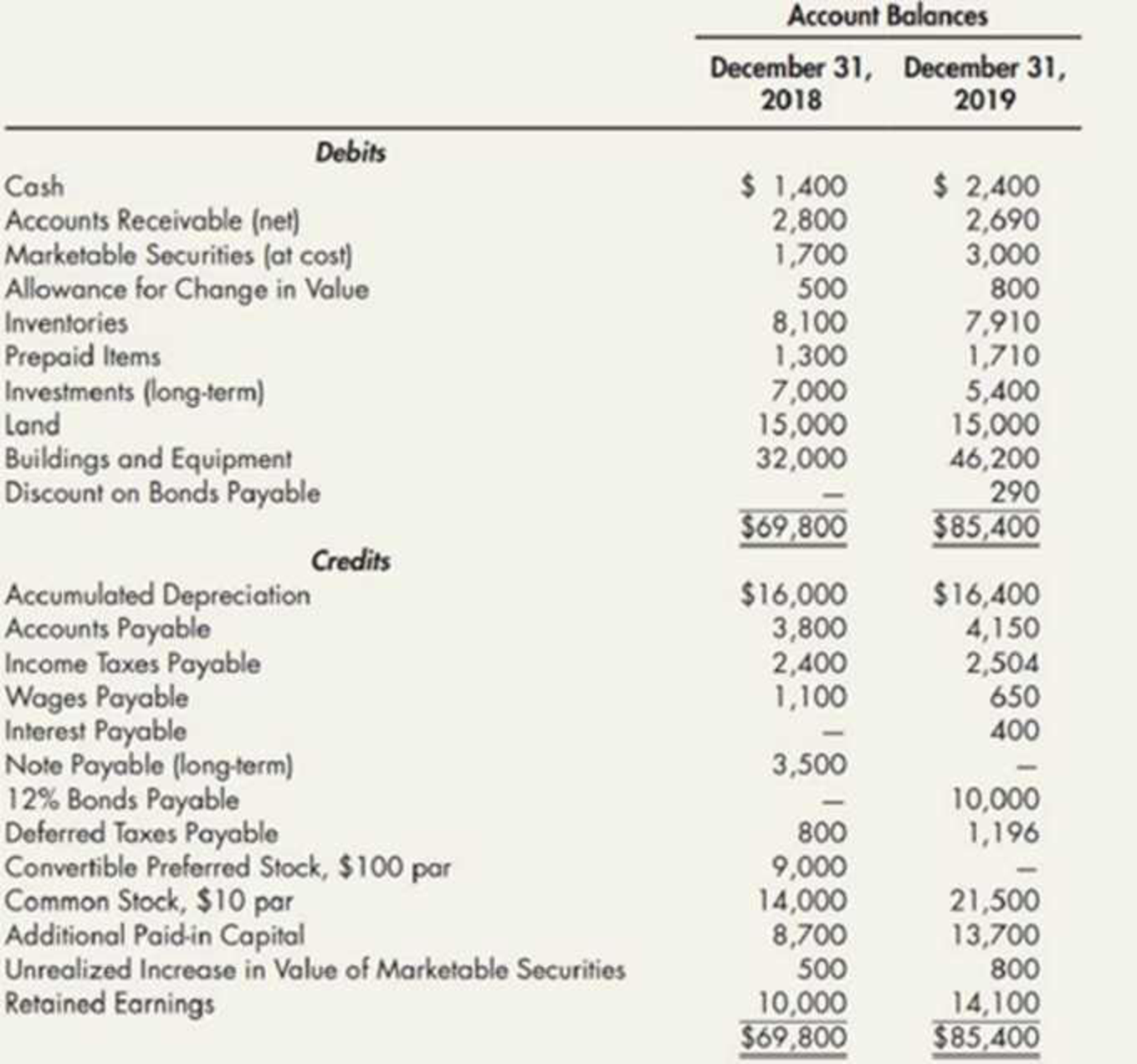Chapter 21, Problem 6P### Intermediate Accounting: Reporting...

3rd Edition
James M. Wahlen + 2 others
ISBN: 9781337788281

#### Solutions

Chapter
Section### Intermediate Accounting: Reporting...

3rd Edition
James M. Wahlen + 2 others
ISBN: 9781337788281
Textbook Problem
1 views

# Spreadsheet and Statement of Cash Flows The following information was taken from Lambcrson Company’s accounting records:1.

To determine

Prepare a statement of cash flows using spreadsheet method.

Explanation

Statement of cash flows: This statement reports all the cash transactions which are responsible for inflow and outflow of cash, and result of these transactions is reported as ending balance of cash at the end of reported period. Statement of cash flows includes the changes in cash balance due to operating, investing, and financing activities.

Worksheet: A worksheet is a spreadsheet used while preparing a financial statement. It is a type of form having multiple columns and it is used in the adjustment process. The use of a worksheet is optional for any organization. A worksheet can neither be considered as a journal nor a part of the general ledger.

Prepare a statement of cash flows using spreadsheet method.

Table (1)

Table (1)

Notes:

a. Depreciation for the year ended is $2,100 b. Calculate accounts receivable. Decrease in accounts receivable = (opening accounts receivable – closing accounts receivable) =$2,800- $2,690=$110

c. Calculate the marketable securities.

Markatable Securities=(Beginning year's markatable securities- Ending year's marketable securities)=$1,700$3,000=$1,300 d. Calculate the decrease in inventories. Decrease in Inventories=(Beginning year's inventories-Ending year's inventories)=$8,100$7,910=$110

e. Calculate the prepaid items.

Prepaid items=(Beginning year's prepaid itemsEnding year's prepaid items )=$1,300$1,710=$410 f. Calculate the increase in accounts payable. Increase in Accounts Payable = (Closing balance of accounts payableOpening balance of accounts payable)=$3,800$4,150=$350

g. Calculate the income taxes payable.

Income Taxes Payable=(Beginning year's income taxes payableEnding year's income taxes payable)=$2,400$2,504=$104 h. Calculate the wages payable. Decrease in Wages Payable=(Beginning year's wages payable-Ending year's wages payable)=$1,100$650=$450

j. Interest payable for the year ended is $400. k. Calculate the retained earnings. Retained Earnings=(Beginning year's retained earnings Ending year's retained earnings)=$10,000$14,100=$4,100

l-1. Calculate the issuance of common stock to preferred stock.

Issuance of common stock = Number of share×Value of share=500×$10=$5000

(Issuance of common stock to preferred stock)=(Issuance of common stock+Additional paid-in capital)=$5,000+$4,000=$9,000 Additional Paid-in Capital=(Beginning year's paid-in capital- Ending year's paid-in capital)=$8,700$13,700=$4,000

1-2

2.

To determine

Prepare the statement of cash flows of L Company for the year ended December 31, 2016.

3.

To determine

Determine the difference in cash flow from operation to sales ratio and profit margin ratio of L Company for the year 2016.

### Still sussing out bartleby?

Check out a sample textbook solution.

See a sample solution

#### The Solution to Your Study Problems

Bartleby provides explanations to thousands of textbook problems written by our experts, many with advanced degrees!

Get Started

#### Find more solutions based on key concepts